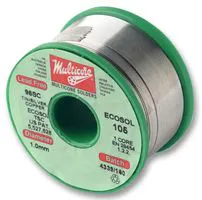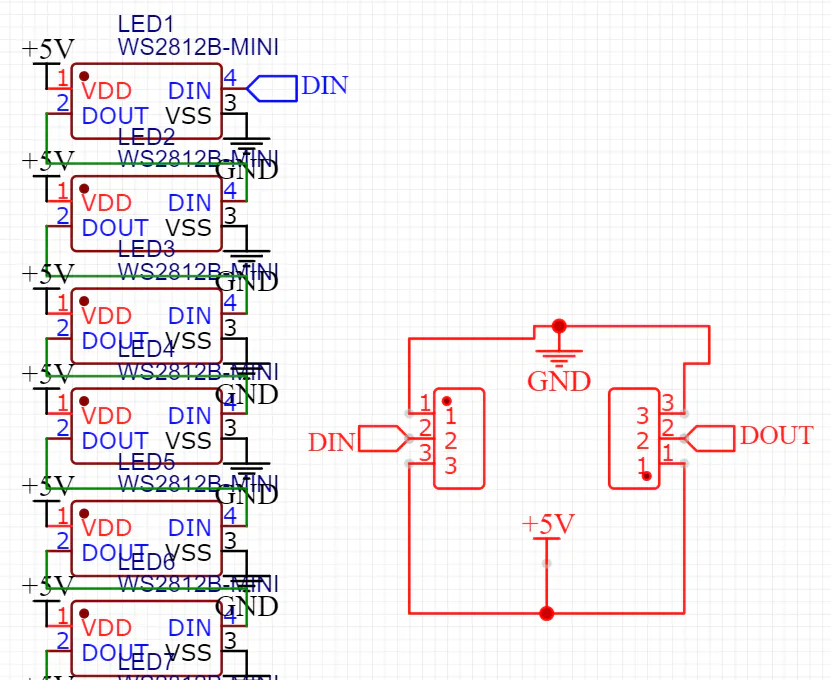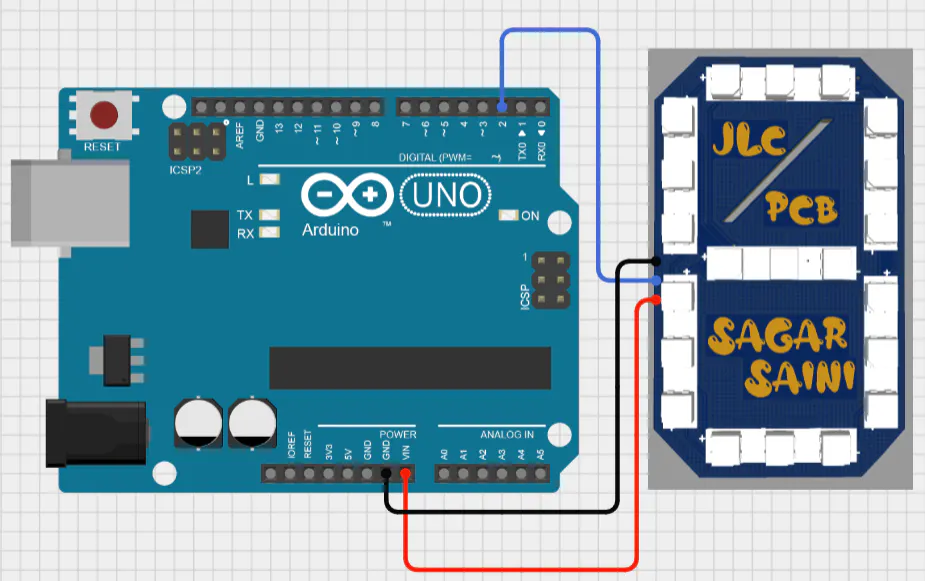# Making 7 segment display using Neo pixel LED

A RGB effect panels which come with addressable led options, controlled using arduino for Project like clock and temp. measurement

IntermediateFull instructions provided1 hour751

## Things used in this project

### Hardware componentsArduino UNO
×1

### Software apps and online servicesJLCPCB EasyEDA

### Hand tools and fabrication machinesSoldering iron (generic)## Schematics

### circuit diagram### Connection## Code

### test code

Arduino
```#include <Adafruit_NeoPixel.h>

#define PIXELS_PER_SEGMENT  3     // Number of LEDs in each Segment
#define PIXELS_DIGITS       1     // Number of connected Digits
#define PIXELS_PIN          2     // GPIO Pin

Adafruit_NeoPixel strip = Adafruit_NeoPixel(PIXELS_PER_SEGMENT * 7 * PIXELS_DIGITS, PIXELS_PIN, NEO_GRB + NEO_KHZ800);

//Pixel Arrangement
/*
a
f   b
g
e   c
d
*/

// Segment array
byte segments = {
//abcdefg
0b0000001,     // Segment g
0b0000100,     // Segment e
0b0001000,     // Segment d
0b0010000,     // Segment c
0b0100000,     // Segment b
0b1000000,     // Segment a
0b0000010     // Segment f
};

//Digits array
byte digits = {
//abcdefg
0b1111110,     // 0
0b0110000,     // 1
0b1101101,     // 2
0b1111001,     // 3
0b0110011,     // 4
0b1011011,     // 5
0b1011111,     // 6
0b1110000,     // 7
0b1111111,     // 8
0b1110011      // 9
};

//Clear all the Pixels
void clearDisplay() {
for (int i = 0; i < strip.numPixels(); i++) {
strip.setPixelColor(i, strip.Color(0, 0, 0));
}
strip.show();
}

void setup() {
strip.begin();
}

void loop() {
//disp_Seg(200);                // Cycle through all segments        (DelayTime)
disp_Digits(1000);            // Show digits from 0-9              (DelayTime)
//disp_Animation();             // Show some Animations with the segments
//disp_CountUP(500, 450);       // Count numbers in Ascending order  (NUMBER, DelayTime)
//  disp_CountDOWN(500, 250);     // Count numbers in Descending order (NUMBER, DelayTime)
}

void disp_Seg(int wait) {
clearDisplay();
for (int d = 0; d < 5; d++) {
for (int i = 6; i > 0; i--) {
for (int n = 0; n < PIXELS_DIGITS; n++) {
writeSegment(n, i);
}
strip.show();
delay(wait);
}
}
}

void disp_Digits(int wait) {
clearDisplay();
for (int i = 0; i < 10; i++) {
for (int n = 0; n < PIXELS_DIGITS; n++) {
writeDigit(n, i);
}
strip.show();
delay(wait);
}
}

void disp_CountUP(int num, int wait) {
clearDisplay();
for (int i = 0; i <= num; i++) {
writeDigit(0, (i / 100) % 10);
writeDigit(1, (i / 10) % 10);
writeDigit(2, (i / 1) % 10);
strip.show();
delay(wait);
}
}

void disp_CountDOWN(int num, int wait) {
clearDisplay();
for (int i = num; i >= 0; i--) {
writeDigit(0, (i / 100) % 10);
writeDigit(1, (i / 10) % 10);
writeDigit(2, (i / 1) % 10);
strip.show();
delay(wait);
}
}

void disp_Animation() {
clearDisplay();
//UP-DOWN
for (int i = 0; i < 7; i++) {
for (int n = 0; n < PIXELS_DIGITS; n++) writeSegment(n, 5);
strip.show();
delay(100);
for (int n = 0; n < PIXELS_DIGITS; n++) writeSegment(n, 0);
strip.show();
delay(100);
for (int n = 0; n < PIXELS_DIGITS; n++) writeSegment(n, 2);
strip.show();
delay(100);
for (int n = 0; n < PIXELS_DIGITS; n++) writeSegment(n, 0);
strip.show();
delay(100);
for (int n = 0; n < PIXELS_DIGITS; n++) writeSegment(n, 5);
strip.show();
delay(100);
}
//LEFT-RIGHT
for (int i = 0; i < 5; i++) {
for (int n = 0; n < PIXELS_DIGITS; n++) {
writeSegment(n, 6);
strip.show();
delay(150);
}
for (int n = PIXELS_DIGITS - 1; n >= 0; n--) {
writeSegment(n, 3);
strip.show();
delay(150);
}
clearDisplay();
for (int n = 0; n < PIXELS_DIGITS; n++) {
writeSegment(n, 1);
strip.show();
delay(150);
}
for (int n = PIXELS_DIGITS - 1; n >= 0; n--) {
writeSegment(n, 4);
strip.show();
delay(150);
}
clearDisplay();
}
//ZIG-ZAG
for (int i = 0; i < 5; i++) {
for (int n = 0; n < PIXELS_DIGITS; n++) {
writeSegment(n, 6);
strip.show();
delay(125);
clearDisplay();
writeSegment(n, 1);
strip.show();
delay(125);
clearDisplay();
writeSegment(n, 4);
strip.show();
delay(125);
clearDisplay();
writeSegment(n, 3);
strip.show();
delay(125);
clearDisplay();
}
}
}

void writeDigit(int index, int val) {
byte digit = digits[val];
for (int i = 6; i >= 0; i--) {
int offset = index * (PIXELS_PER_SEGMENT * 7) + i * PIXELS_PER_SEGMENT;
uint32_t color;
if (digit & 0x01 != 0) {
if (val == 1) color = strip.Color(50, 0,  0);
if (val == 2) color = strip.Color(50, 50, 0);
if (val == 3) color = strip.Color(50, 0, 50);
if (val == 4) color = strip.Color(0, 50,  0);
if (val == 5) color = strip.Color(0, 50, 50);
if (val == 6) color = strip.Color(0,  0, 50);
if (val == 7) color = strip.Color(50, 25, 0);
if (val == 8) color = strip.Color(25, 5, 75);
if (val == 9) color = strip.Color(75, 25, 5);
if (val == 0) color = strip.Color(5, 75, 25);
}
else
color = strip.Color(0, 0, 0);

for (int j = offset; j < offset + PIXELS_PER_SEGMENT; j++) {
strip.setPixelColor(j, color);
}
digit = digit >> 1;
}
}

void writeSegment(int index, int val) {
byte seg = segments[val];
for (int i = 6; i >= 0; i--) {
int offset = index * (PIXELS_PER_SEGMENT * 7) + i * PIXELS_PER_SEGMENT;
uint32_t color;
if (seg & 0x01 != 0) {
if (val == 0) color = strip.Color(50, 0, 0);
if (val == 1) color = strip.Color(0, 50, 50);
if (val == 2) color = strip.Color(0, 50, 0);
if (val == 3) color = strip.Color(50, 0, 50);
if (val == 4) color = strip.Color(50, 50, 50);
if (val == 5) color = strip.Color(0, 0, 50);
if (val == 6) color = strip.Color(50, 50, 0);
}
else
color = strip.Color(0, 0, 0);

for (int j = offset; j < offset + PIXELS_PER_SEGMENT; j++) {
strip.setPixelColor(j, color);
}
seg = seg >> 1;
}
}
```

## Credits

### sagar saini

46 projects • 27 followers
I am Sagar Saini. A PCB engineer and project manager in Farm Network Group.